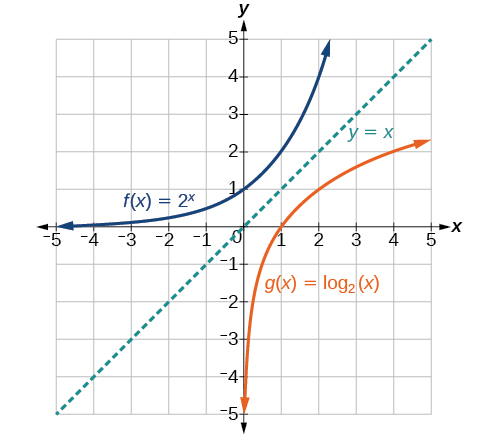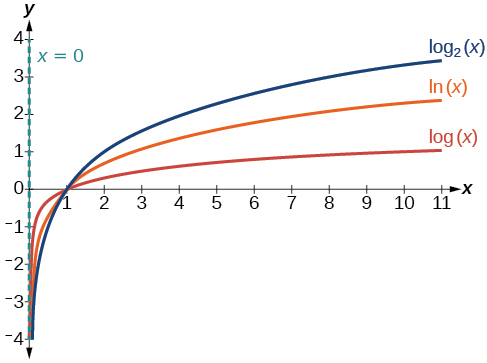# 4.4 Graphs of logarithmic functions  (Page 2/8)

 Page 2 / 8

Given a logarithmic function, identify the domain.

1. Set up an inequality showing the argument greater than zero.
2. Solve for $\text{\hspace{0.17em}}x.$
3. Write the domain in interval notation.

## Identifying the domain of a logarithmic shift

What is the domain of $\text{\hspace{0.17em}}f\left(x\right)={\mathrm{log}}_{2}\left(x+3\right)?$

The logarithmic function is defined only when the input is positive, so this function is defined when $\text{\hspace{0.17em}}x+3>0.\text{\hspace{0.17em}}$ Solving this inequality,

The domain of $\text{\hspace{0.17em}}f\left(x\right)={\mathrm{log}}_{2}\left(x+3\right)\text{\hspace{0.17em}}$ is $\text{\hspace{0.17em}}\left(-3,\infty \right).$

What is the domain of $\text{\hspace{0.17em}}f\left(x\right)={\mathrm{log}}_{5}\left(x-2\right)+1?$

$\left(2,\infty \right)$

## Identifying the domain of a logarithmic shift and reflection

What is the domain of $\text{\hspace{0.17em}}f\left(x\right)=\mathrm{log}\left(5-2x\right)?$

The logarithmic function is defined only when the input is positive, so this function is defined when $\text{\hspace{0.17em}}5–2x>0.\text{\hspace{0.17em}}$ Solving this inequality,

The domain of $\text{\hspace{0.17em}}f\left(x\right)=\mathrm{log}\left(5-2x\right)\text{\hspace{0.17em}}$ is $\text{\hspace{0.17em}}\left(–\infty ,\frac{5}{2}\right).$

What is the domain of $\text{\hspace{0.17em}}f\left(x\right)=\mathrm{log}\left(x-5\right)+2?$

$\left(5,\infty \right)$

## Graphing logarithmic functions

Now that we have a feel for the set of values for which a logarithmic function is defined, we move on to graphing logarithmic functions. The family of logarithmic functions includes the parent function $\text{\hspace{0.17em}}y={\mathrm{log}}_{b}\left(x\right)\text{\hspace{0.17em}}$ along with all its transformations: shifts, stretches, compressions, and reflections.

We begin with the parent function $\text{\hspace{0.17em}}y={\mathrm{log}}_{b}\left(x\right).\text{\hspace{0.17em}}$ Because every logarithmic function of this form is the inverse of an exponential function with the form $\text{\hspace{0.17em}}y={b}^{x},$ their graphs will be reflections of each other across the line $\text{\hspace{0.17em}}y=x.\text{\hspace{0.17em}}$ To illustrate this, we can observe the relationship between the input and output values of $\text{\hspace{0.17em}}y={2}^{x}\text{\hspace{0.17em}}$ and its equivalent $\text{\hspace{0.17em}}x={\mathrm{log}}_{2}\left(y\right)\text{\hspace{0.17em}}$ in [link] .

 $x$ $-3$ $-2$ $-1$ $0$ $1$ $2$ $3$ ${2}^{x}=y$ $\frac{1}{8}$ $\frac{1}{4}$ $\frac{1}{2}$ $1$ $2$ $4$ $8$ ${\mathrm{log}}_{2}\left(y\right)=x$ $-3$ $-2$ $-1$ $0$ $1$ $2$ $3$

Using the inputs and outputs from [link] , we can build another table to observe the relationship between points on the graphs of the inverse functions $\text{\hspace{0.17em}}f\left(x\right)={2}^{x}\text{\hspace{0.17em}}$ and $\text{\hspace{0.17em}}g\left(x\right)={\mathrm{log}}_{2}\left(x\right).\text{\hspace{0.17em}}$ See [link] .

 $f\left(x\right)={2}^{x}$ $\left(-3,\frac{1}{8}\right)$ $\left(-2,\frac{1}{4}\right)$ $\left(-1,\frac{1}{2}\right)$ $\left(0,1\right)$ $\left(1,2\right)$ $\left(2,4\right)$ $\left(3,8\right)$ $g\left(x\right)={\mathrm{log}}_{2}\left(x\right)$ $\left(\frac{1}{8},-3\right)$ $\left(\frac{1}{4},-2\right)$ $\left(\frac{1}{2},-1\right)$ $\left(1,0\right)$ $\left(2,1\right)$ $\left(4,2\right)$ $\left(8,3\right)$

As we’d expect, the x - and y -coordinates are reversed for the inverse functions. [link] shows the graph of $\text{\hspace{0.17em}}f\text{\hspace{0.17em}}$ and $\text{\hspace{0.17em}}g.$Notice that the graphs of   f ( x ) = 2 x   and   g ( x ) = log 2 ( x )   are reflections about the line   y = x .

Observe the following from the graph:

• $f\left(x\right)={2}^{x}\text{\hspace{0.17em}}$ has a y -intercept at $\text{\hspace{0.17em}}\left(0,1\right)\text{\hspace{0.17em}}$ and $\text{\hspace{0.17em}}g\left(x\right)={\mathrm{log}}_{2}\left(x\right)\text{\hspace{0.17em}}$ has an x - intercept at $\text{\hspace{0.17em}}\left(1,0\right).$
• The domain of $\text{\hspace{0.17em}}f\left(x\right)={2}^{x},$ $\left(-\infty ,\infty \right),$ is the same as the range of $\text{\hspace{0.17em}}g\left(x\right)={\mathrm{log}}_{2}\left(x\right).$
• The range of $\text{\hspace{0.17em}}f\left(x\right)={2}^{x},$ $\left(0,\infty \right),$ is the same as the domain of $\text{\hspace{0.17em}}g\left(x\right)={\mathrm{log}}_{2}\left(x\right).$

## Characteristics of the graph of the parent function, f ( x ) = log b ( x )

For any real number $\text{\hspace{0.17em}}x\text{\hspace{0.17em}}$ and constant $\text{\hspace{0.17em}}b>0,$ $b\ne 1,$ we can see the following characteristics in the graph of $\text{\hspace{0.17em}}f\left(x\right)={\mathrm{log}}_{b}\left(x\right):$

• one-to-one function
• vertical asymptote: $\text{\hspace{0.17em}}x=0$
• domain: $\text{\hspace{0.17em}}\left(0,\infty \right)$
• range: $\text{\hspace{0.17em}}\left(-\infty ,\infty \right)$
• x- intercept: $\text{\hspace{0.17em}}\left(1,0\right)\text{\hspace{0.17em}}$ and key point $\left(b,1\right)$
• y -intercept: none
• increasing if $\text{\hspace{0.17em}}b>1$
• decreasing if $\text{\hspace{0.17em}}0

[link] shows how changing the base $\text{\hspace{0.17em}}b\text{\hspace{0.17em}}$ in $\text{\hspace{0.17em}}f\left(x\right)={\mathrm{log}}_{b}\left(x\right)\text{\hspace{0.17em}}$ can affect the graphs. Observe that the graphs compress vertically as the value of the base increases. ( Note: recall that the function $\text{\hspace{0.17em}}\mathrm{ln}\left(x\right)\text{\hspace{0.17em}}$ has base $\text{\hspace{0.17em}}e\approx \text{2}.\text{718.)}$The graphs of three logarithmic functions with different bases, all greater than 1.

#### Questions & Answers

So a horizontal compression by factor of 1/2 is the same as a horizontal stretch by a factor of 2, right?
The center is at (3,4) a focus is at (3,-1), and the lenght of the major axis is 26
The center is at (3,4) a focus is at (3,-1) and the lenght of the major axis is 26 what will be the answer?
Rima
I done know
Joe
What kind of answer is that😑?
Rima
I had just woken up when i got this message
Joe
Can you please help me. Tomorrow is the deadline of my assignment then I don't know how to solve that
Rima
i have a question.
Abdul
how do you find the real and complex roots of a polynomial?
Abdul
@abdul with delta maybe which is b(square)-4ac=result then the 1st root -b-radical delta over 2a and the 2nd root -b+radical delta over 2a. I am not sure if this was your question but check it up
Nare
This is the actual question: Find all roots(real and complex) of the polynomial f(x)=6x^3 + x^2 - 4x + 1
Abdul
@Nare please let me know if you can solve it.
Abdul
I have a question
juweeriya
hello guys I'm new here? will you happy with me
mustapha
The average annual population increase of a pack of wolves is 25.
how do you find the period of a sine graph
Period =2π if there is a coefficient (b), just divide the coefficient by 2π to get the new period
Am
if not then how would I find it from a graph
Imani
by looking at the graph, find the distance between two consecutive maximum points (the highest points of the wave). so if the top of one wave is at point A (1,2) and the next top of the wave is at point B (6,2), then the period is 5, the difference of the x-coordinates.
Am
you could also do it with two consecutive minimum points or x-intercepts
Am
I will try that thank u
Imani
Case of Equilateral Hyperbola
ok
Zander
ok
Shella
f(x)=4x+2, find f(3)
Benetta
f(3)=4(3)+2 f(3)=14
lamoussa
14
Vedant
pre calc teacher: "Plug in Plug in...smell's good" f(x)=14
Devante
8x=40
Chris
Explain why log a x is not defined for a < 0
the sum of any two linear polynomial is what
divide simplify each answer 3/2÷5/4
divide simplify each answer 25/3÷5/12
Momo
how can are find the domain and range of a relations
the range is twice of the natural number which is the domain
Morolake
A cell phone company offers two plans for minutes. Plan A: $15 per month and$2 for every 300 texts. Plan B: $25 per month and$0.50 for every 100 texts. How many texts would you need to send per month for plan B to save you money?
6000
Robert
more than 6000
Robert
For Plan A to reach $27/month to surpass Plan B's$26.50 monthly payment, you'll need 3,000 texts which will cost an additional \$10.00. So, for the amount of texts you need to send would need to range between 1-100 texts for the 100th increment, times that by 3 for the additional amount of texts...
Gilbert
...for one text payment for 300 for Plan A. So, that means Plan A; in my opinion is for people with text messaging abilities that their fingers burn the monitor for the cell phone. While Plan B would be for loners that doesn't need their fingers to due the talking; but those texts mean more then...
Gilbert
can I see the picture
How would you find if a radical function is one to one?
how to understand calculus?
with doing calculus
SLIMANE
Thanks po.
Jenica
Hey I am new to precalculus, and wanted clarification please on what sine is as I am floored by the terms in this app? I don't mean to sound stupid but I have only completed up to college algebra.
I don't know if you are looking for a deeper answer or not, but the sine of an angle in a right triangle is the length of the opposite side to the angle in question divided by the length of the hypotenuse of said triangle.
Marco
can you give me sir tips to quickly understand precalculus. Im new too in that topic. Thanks
Jenica
if you remember sine, cosine, and tangent from geometry, all the relationships are the same but they use x y and r instead (x is adjacent, y is opposite, and r is hypotenuse).
Natalie
it is better to use unit circle than triangle .triangle is only used for acute angles but you can begin with. Download any application named"unit circle" you find in it all you need. unit circle is a circle centred at origine (0;0) with radius r= 1.
SLIMANE
What is domain
johnphilip
the standard equation of the ellipse that has vertices (0,-4)&(0,4) and foci (0, -15)&(0,15) it's standard equation is x^2 + y^2/16 =1 tell my why is it only x^2? why is there no a^2?ByByByByBy